Sudoku: Pincers & Chains

After the simple eliminations are completed in a Sudoku, we sometimes get to a point where the next step isn't obvious.  The Pincer is a pattern that may help eliminate one more candidate and get past the impasse. A Chain is an 'extended' pincer and is a bit harder to detect.  But once you get adept at finding Pincers and Chains, they become invaluable for solving Sudokus.  The basic Pincer pattern is popularly called the 'XY Wing', and chains are called 'XY Chains'.  This note shows a few methods to find Chains.

To get started, perform the simple eliminations and mark each cell with possible remaining candidates.  Now the goal is to eliminate more candidates.  For the basic Pincer, find a sequence of 3 cells, each with only 2 candidates, that have the pattern AB - BC - CA.  Further, the cells containing AB and BC should be in the same zone, and BC and CA should be in a zone.  'Zone' means the same row, column or macro cell.  If you find such a pattern, then other cells that are in the same zone as both AB and CA cannot have the digit A as a candidate.  This is because one of either AB or CA will be the digit A.  Think of AB and CA as the tips of the Pincer and they squeeze out any A that is in its grips.  Applying this technique to cells with 3 candidates is possible, but is more tricky.

Here is a simple example.

 Look at the chain of cells marked green:  b9 - c8 - c6 (or 48 - 58 - 45).  Note that the cell b4 (containing 45) is controlled by both c6 and b9.  The cell c8 can be 5 or 8.  If it is 5 then c6 will be 4 and b4 cannot be 4.  If it is 8 then b9 will be 4 and b4 cannot be 4. Either way, the candidate 4 is eliminated from the purple cell b4 because it is common to b9 (48) and c6 (45).  It is 5. Click on the puzzle to magnify it.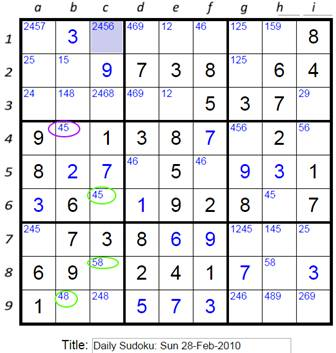In the above example, the green cells b9 and c6 (48 and 45) are both 'controlled' by the green cell c8 (58).  That cell, called the pivot, can either be a 5 or an 8.  If it is 5, then c6 will be 4.  If it is 8 then b9 will be 4.  Either way, one of the pincer tips will be a 4.  The purple cell marked 45 is controlled by both 45 and 48.  Therefore, the purple cell b4 cannot be a 4.

After this elimination, the rest of the puzzle can be rapidly solved.

The trick is to find these Pincers.  Firstly, all the cells that are part of a pincer are those with exactly 2 candidates.  Start with one of them, say AB.  Look for a BC (which has one shared candidate) that is in the same zone.  Then look for a cell containing CA, which is what is left after joining BC & AB and dropping the common B.  If one is found, check if the cells AB and CA control some other common cell, and if that cell has A as a candidate.  If so, eliminate A.

Another simple example:

 The red cells d4 -> d9 -> f7 contain 24 -> 25 -> 45.  The target cell f4  (in purple) cannot be a 4. It becomes 29 and along with the other 29 in e4, it forces the red 24 in d4 to be a 4.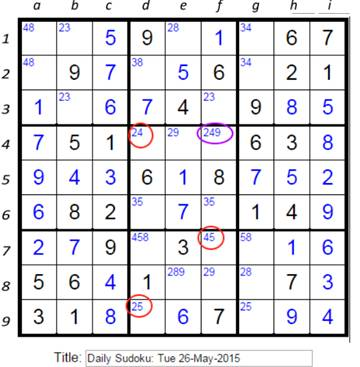Another example, with a rare double yield...

 The cells circled green a2, c3 and d3 force the elimination of 9 from the cells circled in purple d2 & e2.  So they become 5 and 2.  The chain is: 89 - 58 - 59.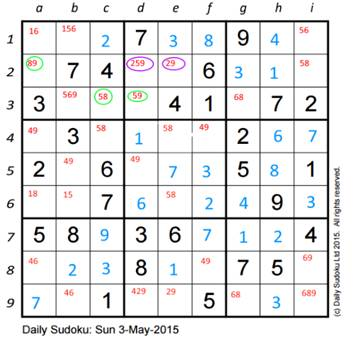Now a game where the Pincer is applied twice in sequence.  First:

 Cells circled in green force the cells circled in purple to be NOT 5.  So purple 35 in e8 becomes 3. The chain is: 75 - 37 - 35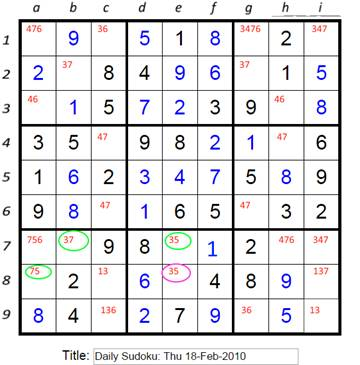Soon, we get to this situation.  The green cells force the purple cell in g3 to be NOT 4.  It becomes 6. The chain is: 46 - 63 - 34 Alternatively, in this case, the pincer could start with c1 (36), then i1 (34) and g3 (46).  Now the 6 in the green circled a3 (46) is eliminated.  Either way...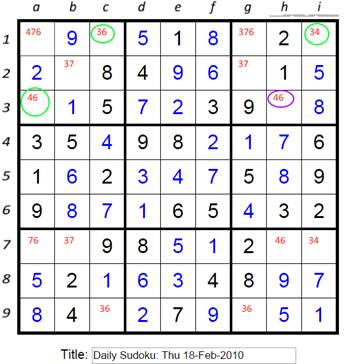Chains

Chains are like longer pincers.  A chain is a sequence of connected pairs where the first and last cell have a common candidate and they control a common cell.  Consider a chain like: AB - BC - CD - DA.  If the two end cells AB and DA can both see a target cell, then the target cannot have an A as a candidate.  This is because if the first cell is A, then the target cannot be A.  If the first cell is B, then the last cell in the chain will be an A, and again the target cannot be A.  The first cell can only be A or B.  This is the same logic used while explaining pincers.

There are many types of chains.  Like Pincers, they are made up of cells what have exactly 2 candidates.  To find a chain like AB - BC - CD - DA, starting with AB, look for a cell with one common candidate, say BC, in the same zone.  Next, from BC, look for a CA in the same zone as BC.  If a CD is found instead, then look for a DA in the same zone as the CD.  If a DE is found instead, then look for a EA in the same zone as the DE.  It seems exhausting, but it becomes intuitive with a little practice.

Here is a simple chain which is easy to recognize because it has 2 reflecting pairs.  In the following, the chain is like: AB - BC - CB - BA.  The end points of the chain control a cell that contains A, which is then eliminated.  View the chain as: 28 - 68 - 68 - 28.

 Here we have a 28 in b6 and a 68 in a5 (circled in red).  We are looking for a 62, but we find another 68 in a9.  The next search is for 28 from a9.  This is 62 & 68 and remove the shared 6 to get 28.  This is found in e9.  Yay! The cell b9 in purple is common to b6 and e9, the ends of the chain.  It cannot be a 2.  That means the cell e9 must be a 2. Note the two blue cells containing 28 could not be used for the chain.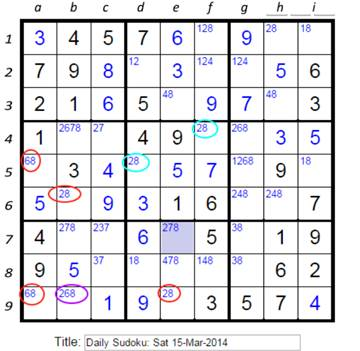Here is another chain: AB - BC - CD - DA.  It eliminates A from cells common to AB and DA.

 Start with a2 (green 59) -> i2 (red 58).  Looking for 89, but find i8 (red 48).  Now looking for 49, which is found in h7 (red 49).  The chain is: 59 -> 58 -> 48 -> 49.  So, a7 (red 569) cannot be a 9.  It becomes a 56. Next, we notice a Pincer the 3 purples starting at a7 -> d7 -> e9  (56 -> 68 -> 58).  This eliminates the two 5s in the bottom row (b9 & c9, circled yellow)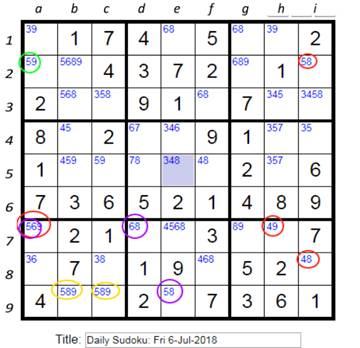Sometimes we find a chain while searching for Pincers.  In Pincers, when we find a potential AB and BC, we search for a suitable cell with candidates CA.  Often we don't find it and continue our search.  Sometimes we then find another pair AD and DC which also needs a CA.  When we note two separate pairs that need the same missing cell, we can try to join them together.  One cell of each pair needs to be share a zone.  The previous example had a common missing link, both 59 & 58, and 49 & 48 needed a 98.  There is no cell with 98 as candidates, but the 2 pairs fit together.

Here is another example of such a chain: AB - BC - CD - DA.  The chain is in green and the affected cell is in red.  The two pairs 69 & 46 and 48 & 89 both needed a 49.

 The chain is: 69 - 46 - 48 - 89 (f3 - f4 - f8 - d7).  The cell d3 circled in red cannot be 9. Think of this as 2 pairs that both need a 94.And here is another...

 Both c1 (78) & b3 (17) and i3 (13) & i9 (38) need a 18.  b3 (17) and i3 (13) connect.  The chain ends are c1 (78) and i9 (38).  They wind up eliminating candidate 8 from i1 in red.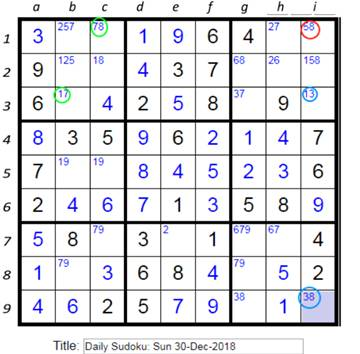In the piece above, we see Green 78 - Green 17 and we search for a 18.  It is just not there.  Then we see Blue 13 - Blue 28 and note that it too needs an 18.  We see that the 17 and 13 share a zone, and walla!, we have a chain that knocks out the 8 from i1 (red 58).  The chain is 78 - 17 - 13 - 38, and it is game over.

The next example has a 'tunnel' connecting two pairs.  The Cells e1 & f2 (red) need a 23. So do b4 & b9 (green).  The two pairs are not directly connected, but there is a way.  Starting with e1 & f2 we are looking for a 23.  We don't see a 23, but there is a 25 in a2.  Moving to a2, we are now looking for a cell with 35.  Moving to a4, we are looking for a 23.  Next move to b4 and look for a 35, and there it is in b9.  The chain is e1 -> f2 -> a2 -> a4 -> b4 -> b9.  The tips e1 and b9 each have a 3.   So the common target cell e9 cannot be a 3.

 Cells e1 & f2 (red) need a 23. So do b4 & b9 (green). They are connect by a 'tunnel' a2 -> a4 -> b4.   They wind up eliminating candidate 3 from e9.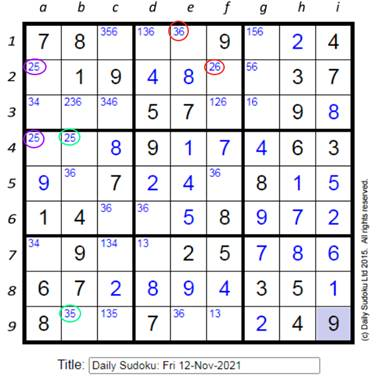## Reflection

Often, there are puzzles where many cells have the same candidate pairs.  It helps to form chains out of them.  These chains look like AB - BA - AB - BA.  I like to mark them with slanted slashes.  Again, this is only for like pairs of candidates.  When a reflected chain is long, we can eliminate  candidates if a cell sees both left & right slashes for the same candidates, it cannot contain those candidate numbers.

The following puzzle has several cells marked 26.  Starting at c3, we mark it with a upward slant.  The matching cells b2 and c8 get an downward slant.  We find that c3 and i9 have opposite slant.  The common cell i3 cannot contain a 2 or a 6 and must be a 5.  The rest is easy.

 Mark chains with matching pairs with slashes, alternating left and right slant, like / and \. Note two 26s circled in red.  They have opposite slant  markings.  Any cell common to them cannot have a 2 or a 6.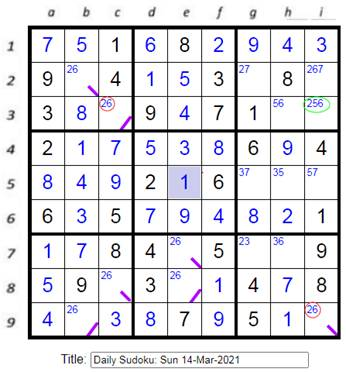The next puzzle also has chains of 26, just a coincidence.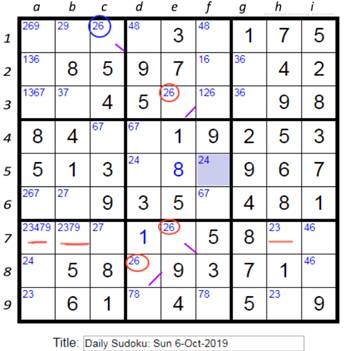The force is strong in this puzzle, but you need to be persistent.  The cells e3, e7 and d8 circled in red are clearly connected. If e3 is 2 then e7 must be 6 and d8 must be 2.  Similarly if e3 is 6, d8 will be equal to e3 and e7 will be opposite.  Marked with right or left slashes (shown in purple).  Cells with the same slant will be equal.

Next, note that there is also a 26 in c1.  In this case it is not too hard to connect it with the chain.  If c1 is a 6, then c7 will be a 2 and so e7 will be a 6.  But if c1 is a 2, c7 is 7, c4 is 6, d4 is 7, d8 is 8, d1 is 4, d5 is 2, d8 is 6 and so e7 will be 2.  In both cases c1 will be the same as e7 and so it should be marked like e7.  We can eliminate 6 as a candidate from a3, that does not do much, but here comes the reward.

Let's look for pincers.  Looking at column 3, c1 and c7 (26 and 27) form a pincer that eliminates 2 in any other cell common to them.  Well, there are no target cells containing a 2.  However, we just showed that c1 is equivalent to e7.  So c7 and e7 cannot allow any 2s.  This eliminates 2 in a7, b7 and h7, the cells marked with a red underline.  The puzzle falls apart easily after this.

One more cool example of reflection, this time from SudokuEssentials.com.  After basic filling, we get the solution below on the left.  We find the pincer marked in red which forces a1 to 2, h1 to 5, h3 to 24 and a4 to 45.  Then the pincer marked in green further reduces i6 to 1, e5 to 1 and b6 to 2.  Now it looks like the figure on the right.  There are many cells with the same two choices.  We can show that cells containing 56  d2 and e6 will be the same and opposite to cells e3 and d5. Also that cells containing 45 a4 and g5 will be the same and b3 will be opposite.  But the jackpot is in the cells containing 24.  Clearly h3 and h8 are opposites.  How about cell i4 ?  If i4 is 2 then cell h8 must also be 2.  If cell h8 is 4, then g7 must be 7, i8 must be 2 and i4 must be 4.  Therefore, cells i4 and h8 will be the same, and opposite to h3.  Mark these 3 cells with green slashes.  Now we see that cells h3 and i4 will always be opposite, so the common cell i3 cannot contain 2 or 4.  Cell i3 must be 7.  The puzzle falls apart easily after this, filling the solver with satisfaction.

It may seem that we did something shady here while showing that cells h8 and i4 will be equal.  We showed that if i4 was 2 then h8 would be 2.  Ideally, we should show that if i4 was 4 then h8 would also be 4.  But we went backwards, showing that if h8 was 4, then i4 would also be 4.  This is ok because it can be proved that if a relation (equal or opposite) is true in one direction for one value, then it must be true for the reverse direction, BUT ONLY IF we can also show that there is a similar relation for the other value as well.

Occasionally, while trying to establish a relation between cells, we may find that a target cell has the same value regardless of the value of the origin cell.  This means that we were sloppy somewhere and missed eliminating a value from the target cell.  Regardless, we got lucky and can lock that value into the target cell.

The lesson here is that it often helps to find related cells that contain like pairs and mark them with slashes.  They are easy to find and can help sometimes.

## Finding long chains

Finding chains is not easy.  Here is one way to do it.  But first, to set the stage, here is a long chain:  18 -> 28 -> 28 -> 38 -> 23 -> 21 (a5 -> e5 -> e9 -> d8 -> f7 -> b7).  The 2 ends 18 & 21 eliminate candidate 1 from b5 and b6, which forces i6 to be 1 and then everything else falls into place.  Later, I found a shorter chain: 18 -> 28 -> 23 -> 23 -> 12, with the same effect.

BTW, not all pincers result in useful eliminations.  There is a pincer 69 -> 16 -> 19 that eliminates the 9 in e1 below, but nothing exciting came out of that.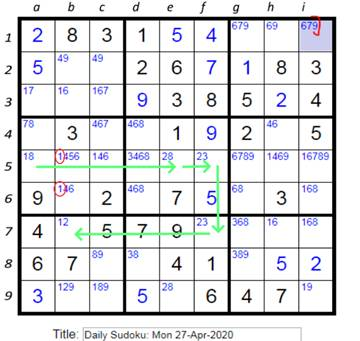This is going to be a brute force search with a way to avoid dead ends.  Say we start with 18 in a5.  Find another cell in the same zone with two candidates, one of which is common to the current cell.  Cell e5 has a 28, where 8 is common to 18 and 28.  Drop the 8 and look for 12 starting from e5.  If there is no 12, then look for a cell with a common candidate.  The 23 in f5 has a 2 that is common to 12 and 23.  Drop the 2 and look for the remaining candidate pair: 13.  There is no 13, but there is a 23 in f7.  Drop the 3 that is common and look for the remaining candidate pair: 12.  Wow, there is one at b7 which completes the chain.  Next, verify that the start and end cells in the chain have a common candidate.  Start cell 18 and 12 share the candidate 1.  Then check if there are any cells common to the start and end cells.  Yup, cells b5 & b6 are common and they both have 1 as a candidate.  Eliminate the 1 from those cells and move on.